International Tables for Crystallography (2011). Vol. A1, ch. 1.7, pp. 57-69https://doi.org/10.1107/97809553602060000796

## Contents

• 1.7. The Bilbao Crystallographic Server  (pp. 57-69)
• 1.7.1. Introduction  (p. 57) | html | pdf |
• 1.7.2. Databases and retrieval tools  (pp. 57-58) | html | pdf |
• 1.7.2.1. Space-group data  (pp. 57-58) | html | pdf |
• 1.7.2.2. Database on maximal subgroups  (p. 58) | html | pdf |
• 1.7.2.2.1. Maximal subgroups of indices 2, 3 and 4 of the space groups  (p. 58) | html | pdf |
• 1.7.2.2.2. Maximal isomorphic subgroups  (p. 58) | html | pdf |
• 1.7.3. Group–subgroup and group–supergroup relations between space groups  (pp. 58-66) | html | pdf |
• 1.7.3.1. Subgroups of space groups  (pp. 58-64) | html | pdf |
• 1.7.3.1.1. The program SUBGROUPGRAPH   (pp. 58-61) | html | pdf |
• 1.7.3.1.2. The program HERMANN   (p. 61) | html | pdf |
• 1.7.3.1.3. The program COSETS   (p. 62) | html | pdf |
• 1.7.3.1.4. The program CELLSUB   (pp. 62-63) | html | pdf |
• 1.7.3.1.5. The program COMMONSUBS   (pp. 63-64) | html | pdf |
• 1.7.3.2. Supergroups of space groups  (pp. 64-66) | html | pdf |
• 1.7.3.2.1. The programs MINSUP and SUPERGROUPS   (pp. 64-66) | html | pdf |
• 1.7.3.2.2. The program CELLSUPER   (p. 66) | html | pdf |
• 1.7.3.2.3. The program COMMONSUPER   (p. 66) | html | pdf |
• 1.7.4. Relations of Wyckoff positions for a group–subgroup pair of space groups  (pp. 66-68) | html | pdf |
• 1.7.4.1. The program WYCKSPLIT   (pp. 67-68) | html | pdf |
• References | html | pdf |
• Figures
• Fig. 1.7.3.1. General contracted graph for(No. 92) >(No. 4) as given by the program SUBGROUPGRAPH   (p. 60) | html | pdf |
• Fig. 1.7.3.2. Contracted graph for the pair of space groups(No. 92) >(No. 4), index 4, as given by the program SUBGROUPGRAPH   (p. 60) | html | pdf |
• Fig. 1.7.3.3. Complete graph for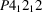(No. 92) >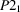(No. 4), index 4, as given by the program SUBGROUPGRAPH   (p. 60) | html | pdf |
• Fig. 1.7.3.4. Group–subgroup graph for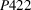(No. 89) >(No. 4), index 8  (p. 61) | html | pdf |
• Fig. 1.7.3.5. Space-group diagrams for ( a )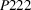, No. 16, with specialized cell metrics (see the text) and ( b ), No. 89  (p. 65) | html | pdf |
• Fig. 1.7.4.1. Sequence of calculations of WYCKSPLIT for the splitting of the Wyckoff positions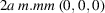and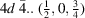of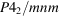, No. 136, with respect to its subgroup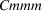, No. 65, of index 2  (p. 67) | html | pdf |
• Tables
• Table 1.7.3.1. Group–subgroup relations for P 4 1 2 1 2 (No. 92) > P 2 1 (No. 4), index 4  (p. 59) | html | pdf |
• Table 1.7.3.2. P 422, No. 89, supergroups of P 222, No. 16 ( a = b = c ), index 2, as determined by MINSUP   (p. 65) | html | pdf |
• Table 1.7.4.1. Wyckoff positions of(No. 65) with multiplicities 2 and 8  (p. 67) | html | pdf |